C++ Constructor Tutorial With Example | Constructors in C++ Explained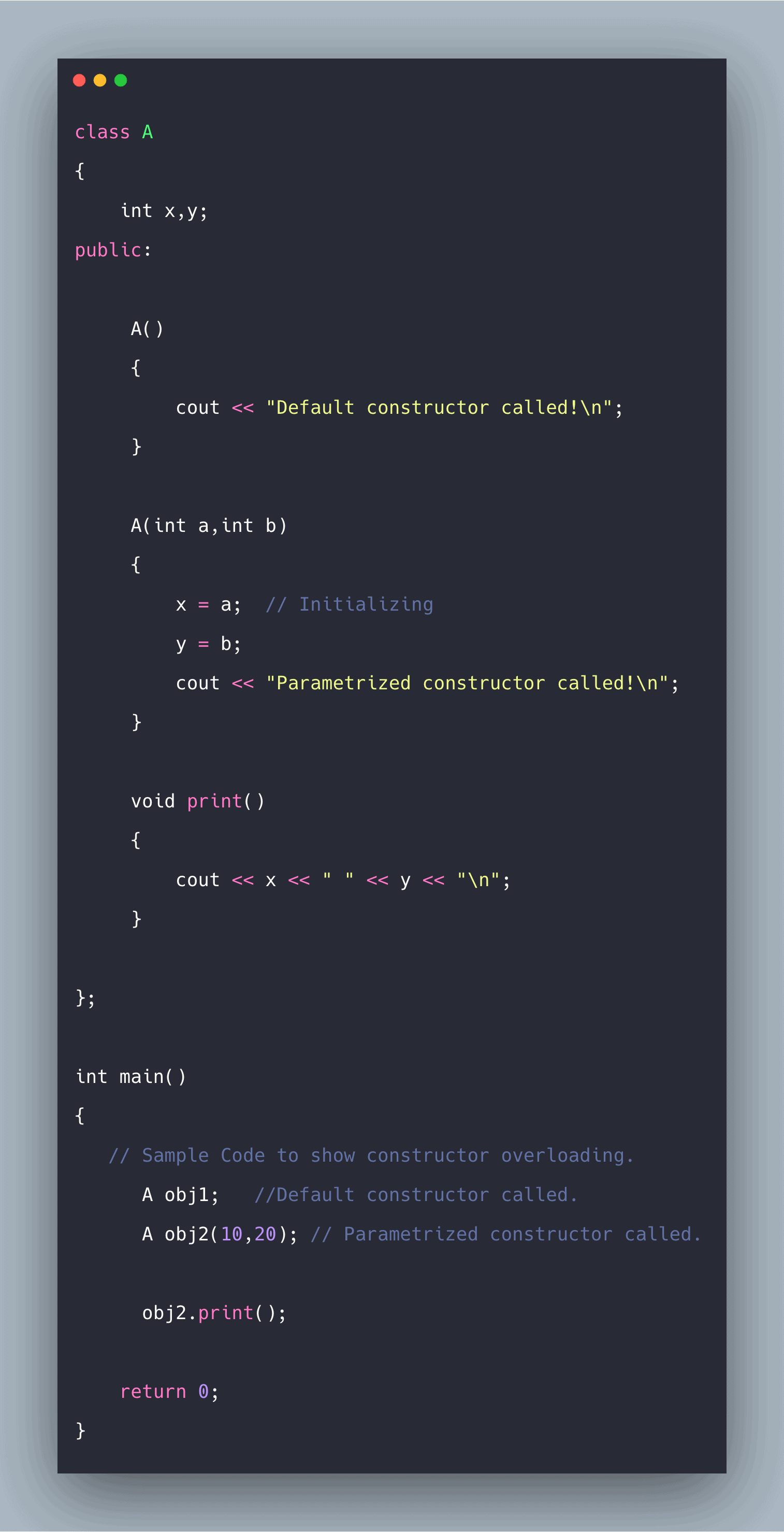C++ Constructor Tutorial With Example | Constructors in C++ is today’s topic. A constructor is associated with classes in C++. As the name suggests, a constructor is used to initializing the member variables of an instance of a class that is the object of a class. A constructor is a particular type of member function that initializes an object automatically when it is created. The compiler identifies a given member function is a constructor by its name and the return type. A constructor has a same name as that of a class, and it does not have any return type. Also, the constructor is always public.

C++ Constructor Tutorial

A constructor is the member function of a class. But they do differ from actual function in the following ways:

1. Constructor have the same name as the class name.
2. The constructor doesn’t have a return type, unlike other member functions.
3. If there is no explicit definition of constructor in a class, a default constructor is automatically called.
4. If we do not specify a constructor, C++ compiler generates a default constructor for us (expects no parameters and has a hollow body).

How constructor works

Whenever an instance of the class is created a member function without any return type having the same name as the class is called automatically. Even if this constructor is not defined explicitly, a default constructor is still called.

Since it is a function, it can take arguments and has function body also. So, arguments can be passed to it by explicitly defining custom constructor, and inside its body, arguments passed can be used to initialize the member variables.

Use of constructors

Constructor, as the name suggests, is usually used to initialize the member variables of the object of a class automatically, whenever an object of that class is created. Suppose there is a class Rectangle that stores length, breadth, and area of the rectangle.

Also, it has some member functions that deal with it. If we want that whenever an object of class Rectangle is created length, breadth and area should get initialized automatically. Then, in this case, the constructor is used to initialize the variables.

Types of constructors in C++

Default constructor

As the name suggests if we have not explicitly defined a constructor while defining the class, a default constructor with no parameters and a hollow body is called.

To check this, we can explicitly write this default constructor with a print statement inside its body to check that it was called. When an instance or object of a class is created, default constructor will be called automatically.

In the code mentioned below when the object ‘obj’ of class A is created default constructor is called automatically, and it is shown by the print function inside the default constructor which outputs to the outstream “Default constructor called!”.

See the following default constructor in c++ code.

``````#include <iostream>
using namespace std;
class A
{
int x,y;
public:
A()
{
cout << "Default constructor called!";
}
};

int main()
{
// Sample Code to show default constructor
A obj; // making a object of class A
return 0;
}
``````

See the below output.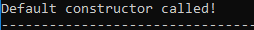Parameterized constructor

Unlike default constructor in the parameterized constructor, we can pass as arguments the values to initialize the member variables of an object of a class.

It is the same like normal function where we pass arguments, and the only difference is that it doesn’t have a return type. It is more like function overloading as we can have a different set of constructors for a different set of input parameters, and this is termed as constructor overloading.

The parameterized constructor can be called in two different ways, namely explicit and implicit.

``````class_name obj = class_name(parameter1,parameter2); // Explicit way
class_name obj(parameter 1,parameter 2); //Implicit way
``````

Both do the same thing that initializes the member variables of the object of a class.

In the code mentioned below, class A contains two member variables x and y. Object obj is created with values 10, and 20 passed to it as arguments. Here, the implicit calling of the parameterized constructor is called. Then inside the body of the parameterized constructor ‘x=a’ and ‘y=b’ is initializing the member variables of the object.

``````#include <iostream>
using namespace std;

class A
{
int x,y;
public:

A()
{
cout << "Default constructor called!";
}

A(int a,int b)
{
x = a;  // Initializing
y = b;
cout << "Parameterized constructor called!n";
}

void print()
{
cout << x << " " << y << "n";
}

};

int main()
{
// Sample Code to show default constructor
A obj(10,20); // making a object of class A   -- >Implicit

obj.print();

return 0;
}
``````

See the following output.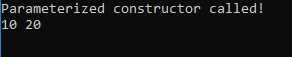Copy Constructor

It is somewhat different from the other two constructors in a way that it is used to initialize the member variables of one object by the values of another object of the same class.

Another object of the same class is passed as a reference in the argument which is then used to initialize the member variables of the concerned object.

It is usually called when a function returns an object, or an object is being passed as an argument to a function. It has the following syntax of defining it.

``class_name( const class_name & object_of_same_class );``

When the old object is passed an argument, the values of the member variables of the old object can be assigned to members of the new object explicitly.

In the code mentioned below, two objects, obj, and obj2, have been created. The first object is just the parameterized constructor used in the above section. obj2  is initialized with the values of the member variables of the object obj by passing obj as an argument while creating object obj2. ‘x=old.x’ and ‘y=old.y’ is assigning the values of the old object to a new object.

``````#include <iostream>
using namespace std;

class A
{
int x,y;
public:

A()
{
cout << "Default constructor called!";
}
A(int a,int b)
{
cout << "Parameterized Constructor called!n";
x=a;
y=b;
}
A(const A & old )
{
// old is the old object being passed
x = old.x;  //This object's x to old object's x
y = old.y;

cout << "Copy Constructor called!n";
}

void print()
{
cout << x << " " << y << "n";
}

};

int main()
{
// Sample Code to show default constructor
A obj(10,20); // making a object of class A   -- >Implicit
A obj2(obj);  // Copy Constructor called old object 'obj' is passed
obj2.print();

return 0;
}
``````

See the below output.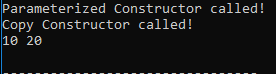Since the constructor is very much similar to a function, constructor overloading is also very similar to function overloading. So, different constructors can be called based on a different set of arguments passed. All the constructors have the same name, which is the same as the class name.

In the code mentioned below, the different constructor is called based on different argument passed. Class A has two constructors one without any arguments that is default one and another one with parameters ‘a’ and ‘b.

Two objects, obj1 and obj2, are created. In obj1 constructor without arguments is called. In obj2 constructor with parameters is called, because parameters ‘10’ and ‘20’ has been passed to it. It sets the member variable of obj2 that is  ‘x=a’ and ‘y=b.’

See the following code.

``````#include <iostream>
using namespace std;

class A
{
int x,y;
public:

A()
{
cout << "Default constructor called!n";
}

A(int a,int b)
{
x = a;  // Initializing
y = b;
cout << "Parametrized constructor called!n";
}

void print()
{
cout << x << " " << y << "n";
}

};

int main()
{
A obj1;   //Default constructor called.
A obj2(10,20); // Parametrized constructor called.

obj2.print();

return 0;
}
``````

See the following output.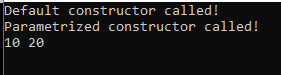Finally, C++ Constructor Tutorial With Example | Constructors in C++ Explained is over.